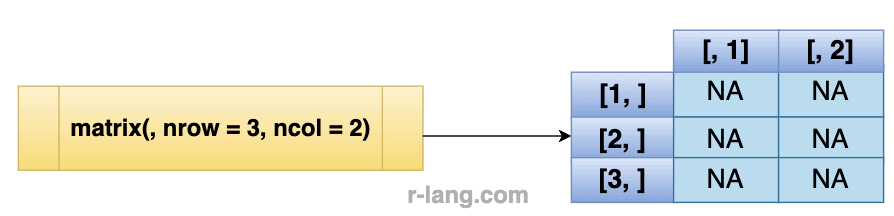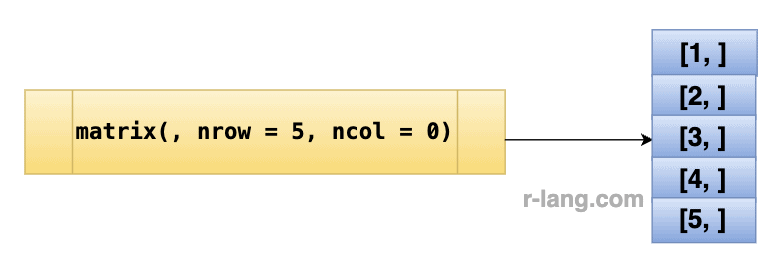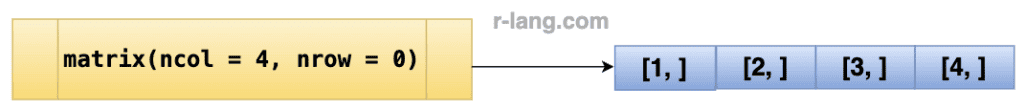# How to Create an Empty Matrix in R

Here are three ways to create an empty matrix in R:

1. Using row and column
2. Using only row
3. Using only column

## Method 1: Using a row and column``````mat <- matrix(, nrow = 3, ncol = 2)

print(mat)``````

Output

``````     [,1]  [,2]
[1,]  NA    NA
[2,]  NA    NA
[3,]  NA    NA``````

We created a 3×2 matrix mat with three rows and two columns, initially filled with NA values because there is no data and it is empty.

## Method 2: Using only a row``````mat <- matrix(, nrow = 5, ncol = 0)

print(mat)``````

Output

``````[1,]
[2,]
[3,]
[4,]
[5,]``````

We created a matrix mat with 5 rows and 0 columns.

## Method 3: Use the column``````mtrx <- matrix(ncol = 4, nrow = 0)

mtrx``````

Output

`` [,1] [,2] [,3] [,4]``

That is it.

## Related posts

Create an empty list

Create an empty vector

Create an empty data frame